Like   Tweet   Pin   +1   in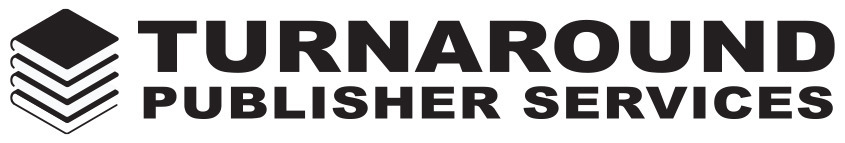table div table+table div table{width:100%;padding:0}table div table+table div table img{width:96.23%;padding:0;float:none}table div table+table div table td{width:100%;padding:0 1.88% 18px}/* styles */## 30th July 2018

 table div table+table+table+table+table div table{width:100%;padding:0}table div table+table+table+table+table div table img{width:96.23%;padding:0;float:none}table div table+table+table+table+table div table td{width:100%;padding:0 1.88% 18px}/* styles */## Akashic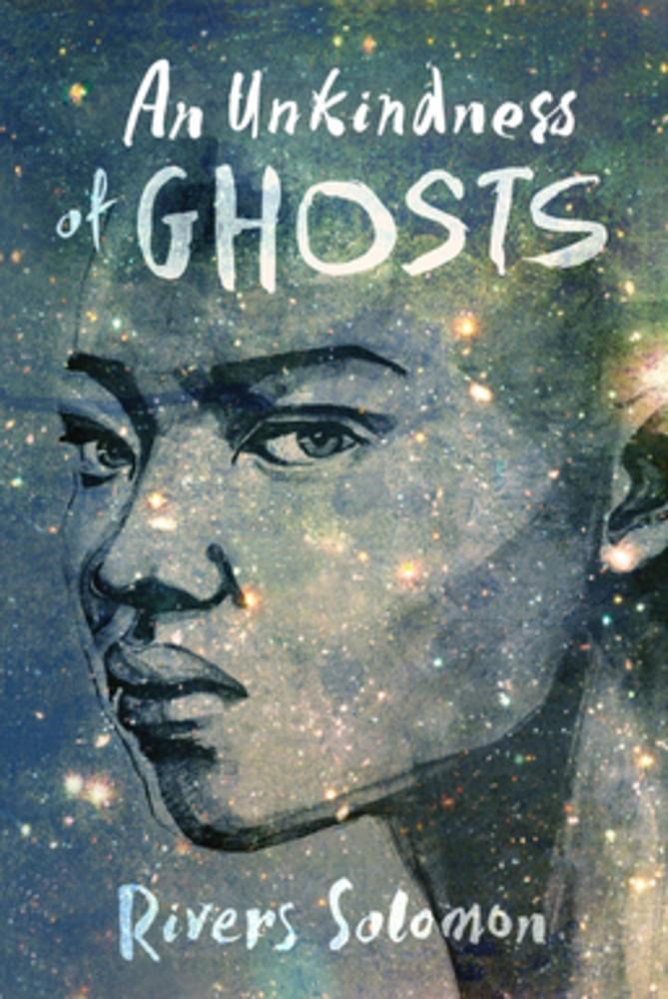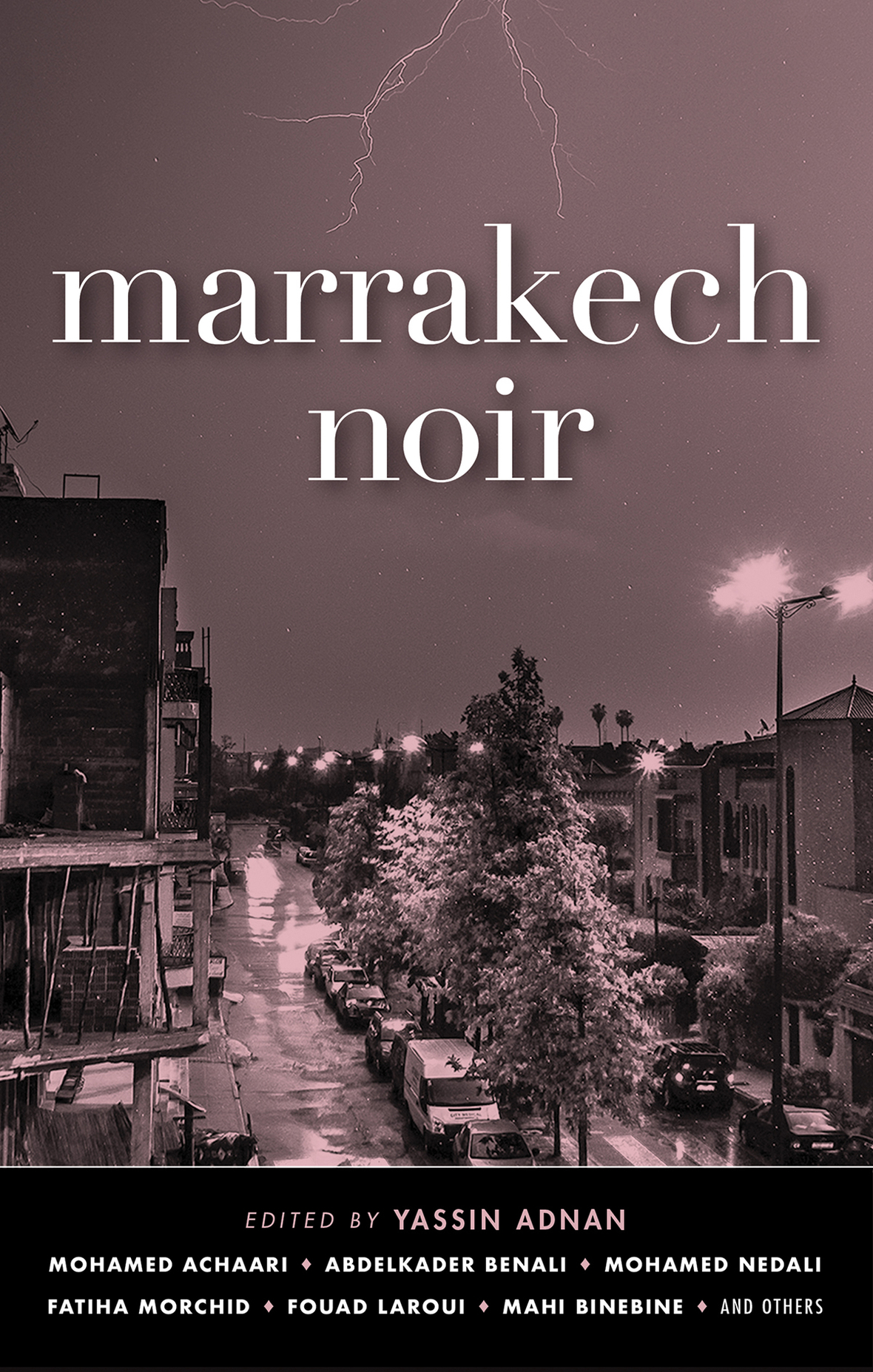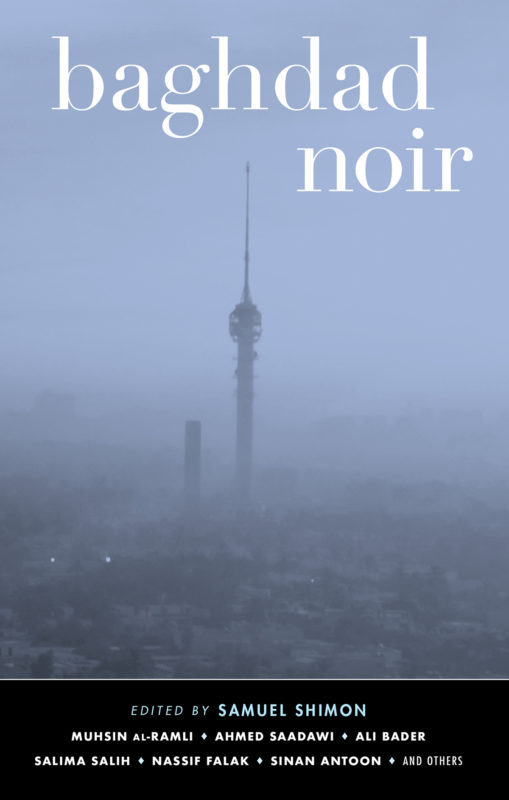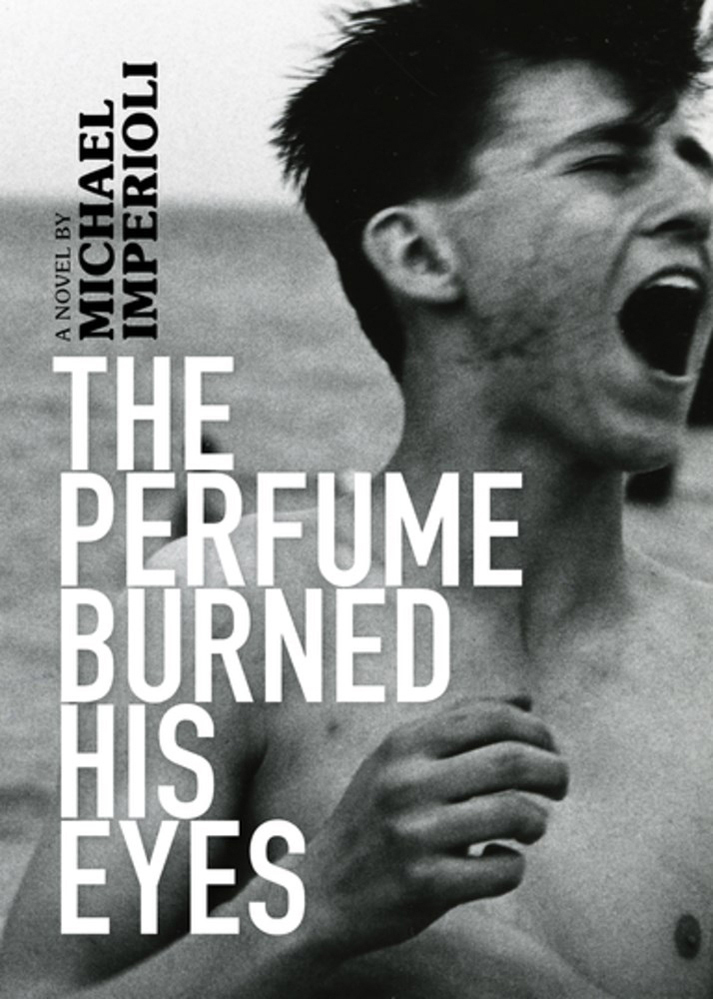/* styles */ Featured books: An Unkindness of Ghosts, £11.99; Marrakech Noir, £11.99; Baghdad Noir, £11.99 & The Perfume Burned His Eyes, £19.99 Rivers Solomon was interviewed about An Unkindness of Ghosts on PBS' Read Awakening as part of their 'Great American Reads' series. The full interview is available here. Rivers was also mentioned in a segment on the evolution of science fiction on PBS' It's Lit!, also part of the 'Great American Reads' series. That's available here. Marrakech Noir and Baghdad Noir were both included in ArabLit's list of 'Five books to look for in July and August 2018' here, and the latter was also covered in the Midwest Book Review. The Perfume Burned His Eyes was reviewed in Santa Barbara Magazine.
 table div table+table+table+table+table+table+table+table+table div table{width:100%;padding:0}table div table+table+table+table+table+table+table+table+table div table img{width:96.23%;padding:0;float:none}table div table+table+table+table+table+table+table+table+table div table td{width:100%;padding:0 1.88% 18px}/* styles */## Reel Art Press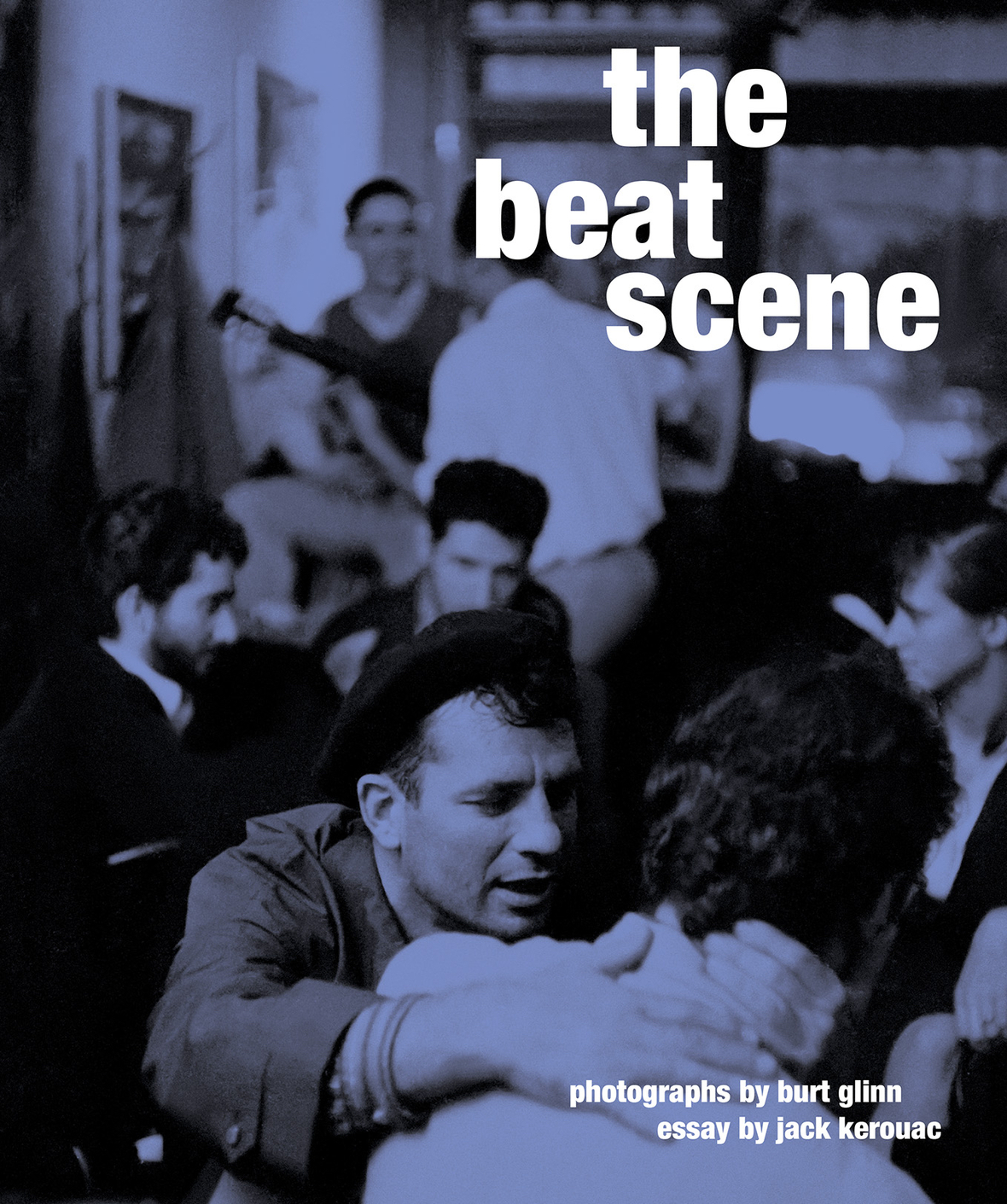Featured book: The Beat Scene, £29.95

Burt Glinn's The Beat Scene was extracted in The Guardian here.

 table div table+table+table+table+table+table+table+table+table+table+table+table div table{width:100%;padding:0}table div table+table+table+table+table+table+table+table+table+table+table+table div table img{width:96.23%;padding:0;float:none}table div table+table+table+table+table+table+table+table+table+table+table+table div table td{width:100%;padding:0 1.88% 18px}/* styles */## Oldcastle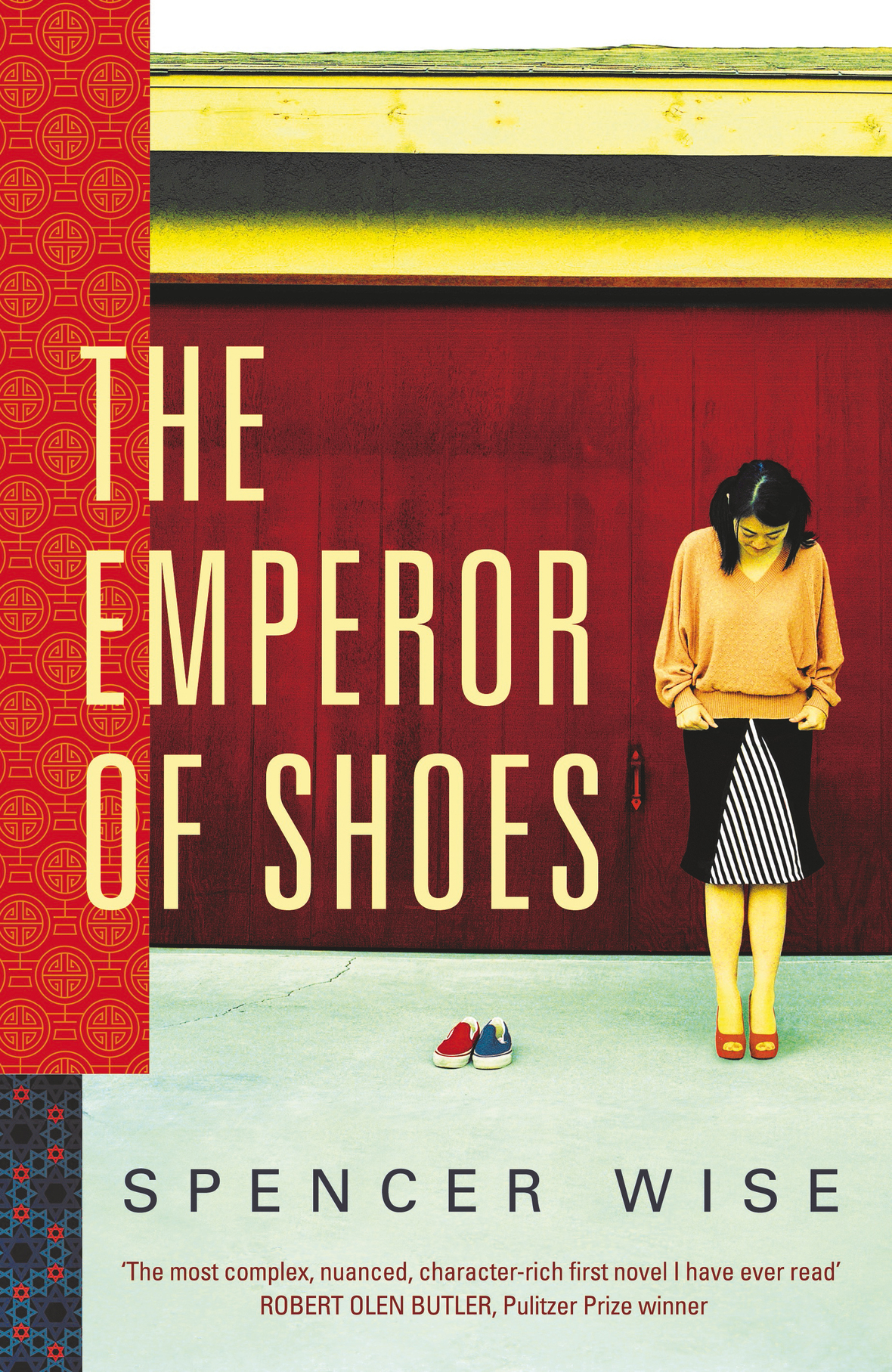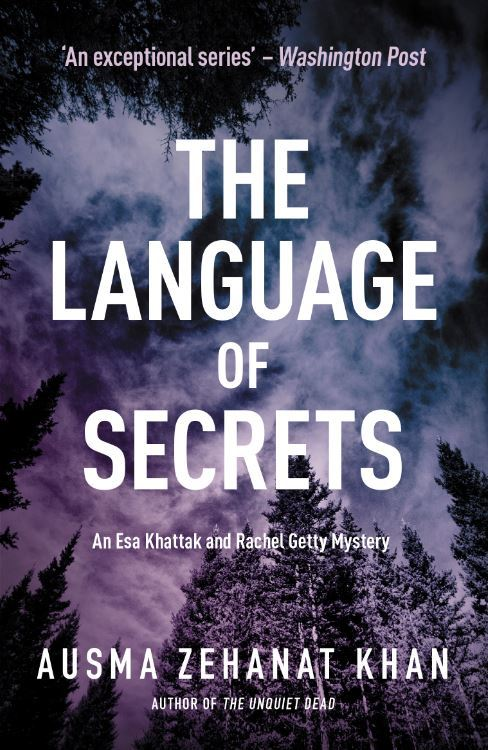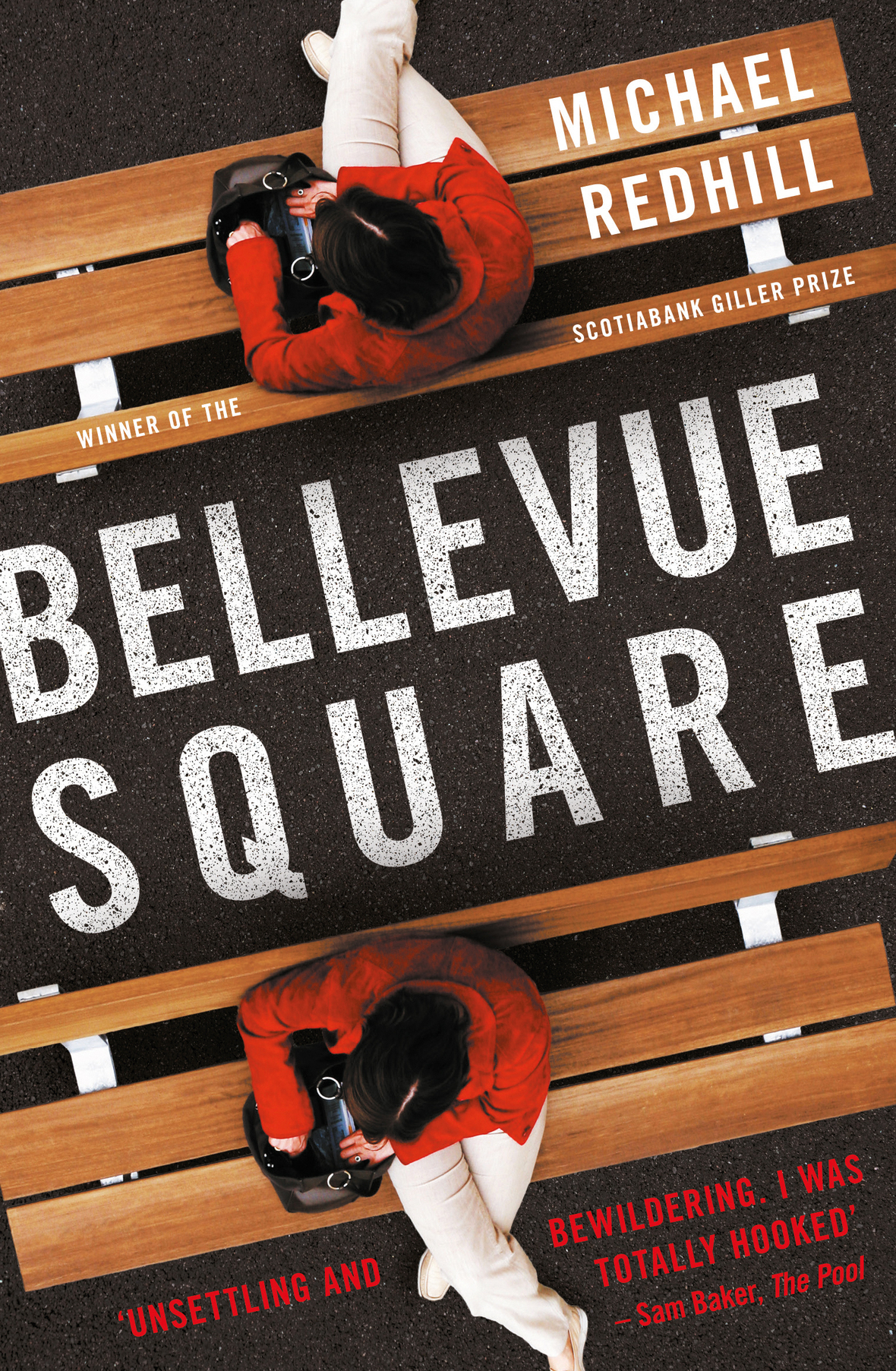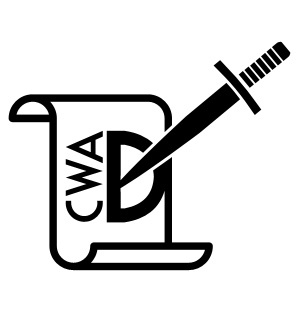/* styles */ Featured books: The Emperor of Shoes, £16.99; The Language of Secrets, £7.99; Bellevue Square, £8.99; The Frozen Woman, £7.99 & Gravesend, £8.99 for the CWA Daggers Awards. Spencer Wise's The Emperor of Shoes landed a total of five reviews this week, including one in the New York Times here. Spencer also wrote a feature for Writing.ie here. The Language of Secrets author Ausma Zehanat Khan also wrote an article for Writing.ie here, while the book was reviewed in four outlets including the Financial Times and Medium. Bellevue Square was recommended in the Guardian's list of 'The Best Recent Thrillers', and was also mentioned by Barry Forshaw on Phil William's BBC Radio 5 Live show. Oldcastle have also had two books nominated for the CWA Daggers Awards: John Michelet's The Frozen Woman and Gravesend by William Boyle. Congratulations to both!
 table div table+table+table+table+table+table+table+table+table+table+table+table+table+table+table+table div table{width:100%;padding:0}table div table+table+table+table+table+table+table+table+table+table+table+table+table+table+table+table div table img{width:96.23%;padding:0;float:none}table div table+table+table+table+table+table+table+table+table+table+table+table+table+table+table+table div table td{width:100%;padding:0 1.88% 18px}/* styles */## Text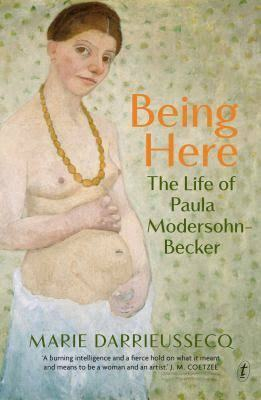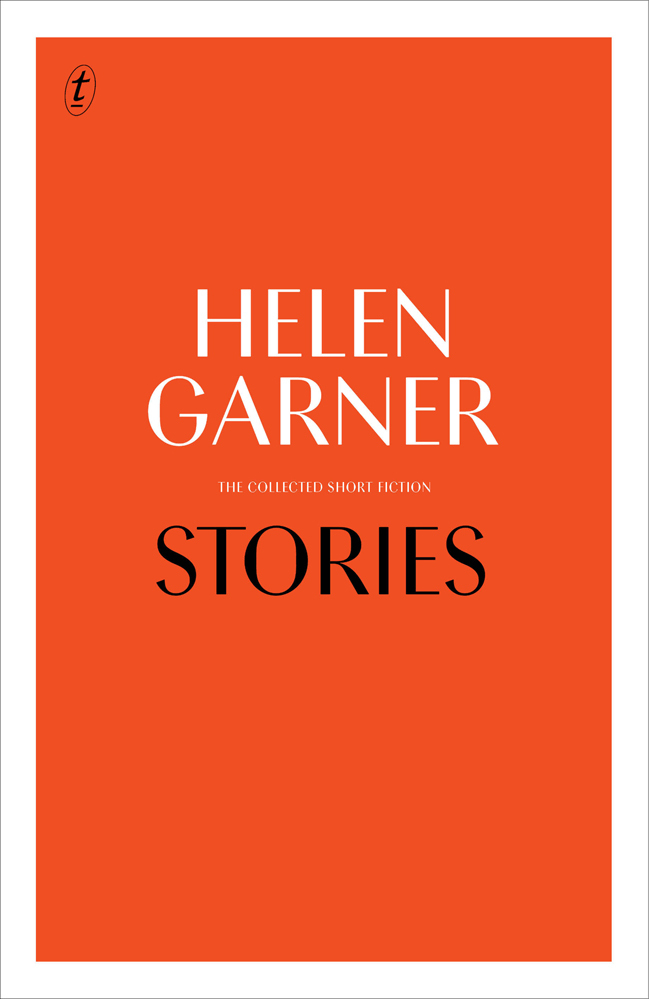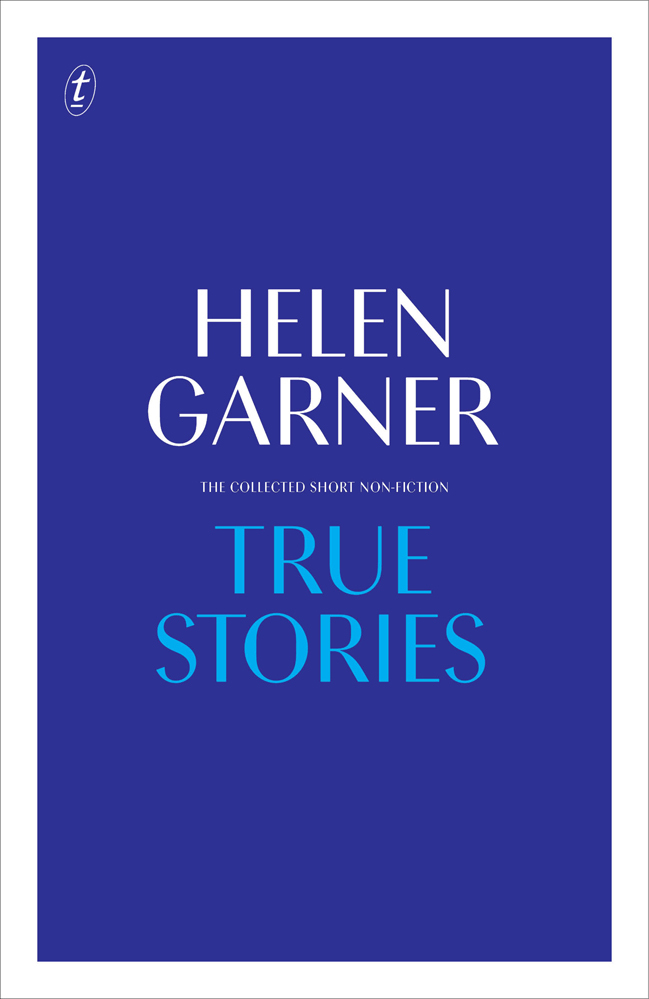/* styles */ Featured books: Being Here, £12.99; Stories: Collected Short Fiction, £14.99 & True Stories: Collected Non-Fiction, £25.00 Being Here by Marie Darrieussecq was mentioned in a graphic article in the New Yorker by Lauren Weinstein. Meanwhile, Helen Garner's collected works Stories and True Stories were featured in an article in the TLS available here.
 table div table+table+table+table+table+table+table+table+table+table+table+table+table+table+table+table+table+table+table+table div table{width:100%;padding:0}table div table+table+table+table+table+table+table+table+table+table+table+table+table+table+table+table+table+table+table+table div table img{width:96.23%;padding:0;float:none}table div table+table+table+table+table+table+table+table+table+table+table+table+table+table+table+table+table+table+table+table div table td{width:100%;padding:0 1.88% 18px}/* styles */## Seven Stories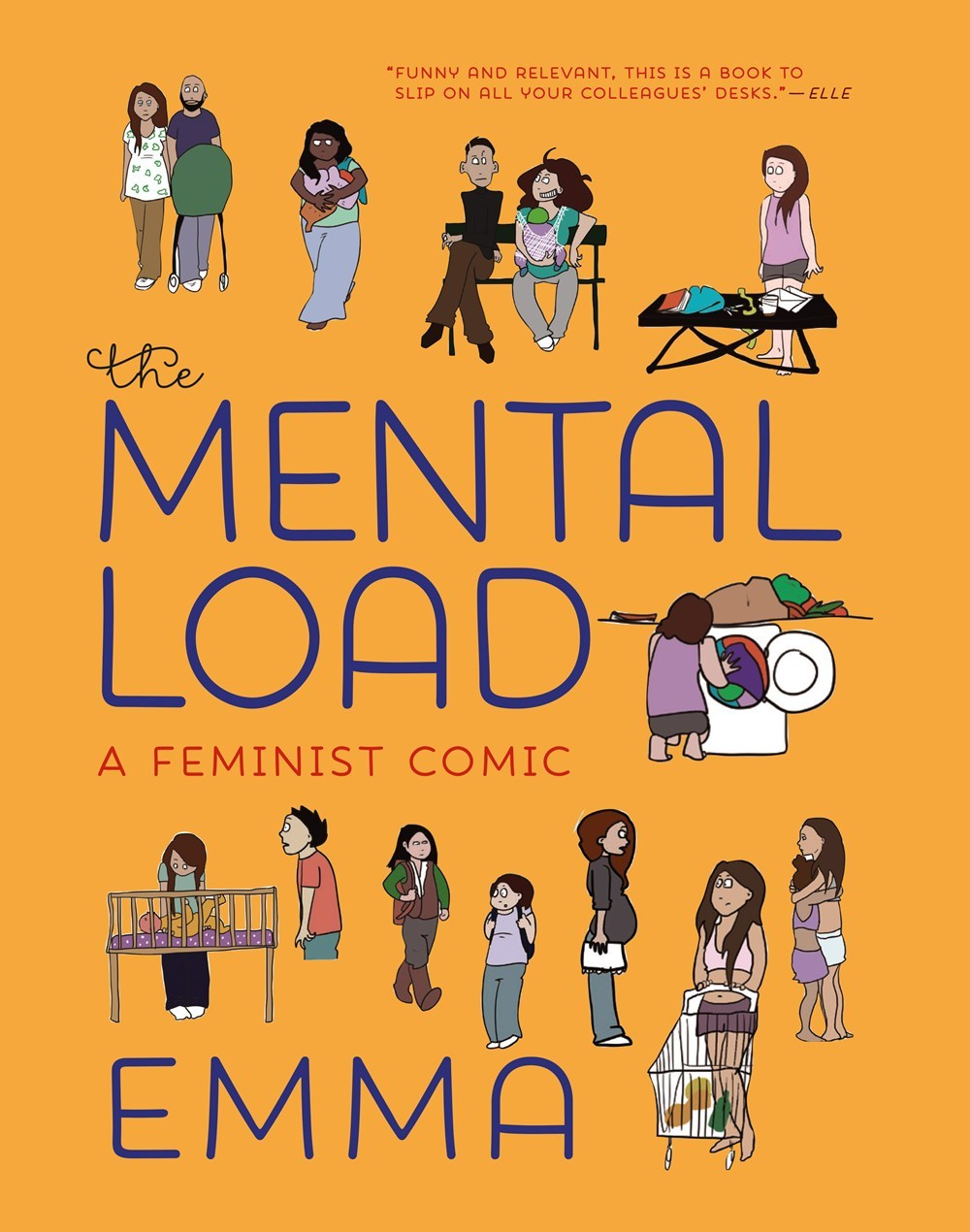Featured book: The Mental Load, £12.99

Jacky Fleming has described The Mental Load as 'visual essays which are transformative agents of change,' while Bridget Schulte called the book 'an eye-opening gem.'

 table div table+table+table+table+table+table+table+table+table+table+table+table+table+table+table+table+table+table+table+table+table+table+table div table{width:100%;padding:0}table div table+table+table+table+table+table+table+table+table+table+table+table+table+table+table+table+table+table+table+table+table+table+table div table img{width:96.23%;padding:0;float:none}table div table+table+table+table+table+table+table+table+table+table+table+table+table+table+table+table+table+table+table+table+table+table+table div table td{width:100%;padding:0 1.88% 18px}/* styles */## Europa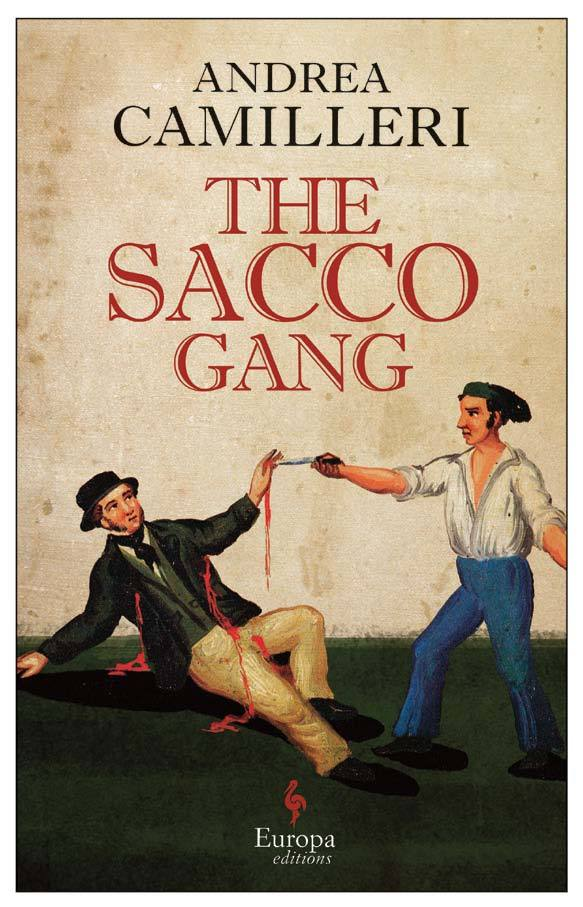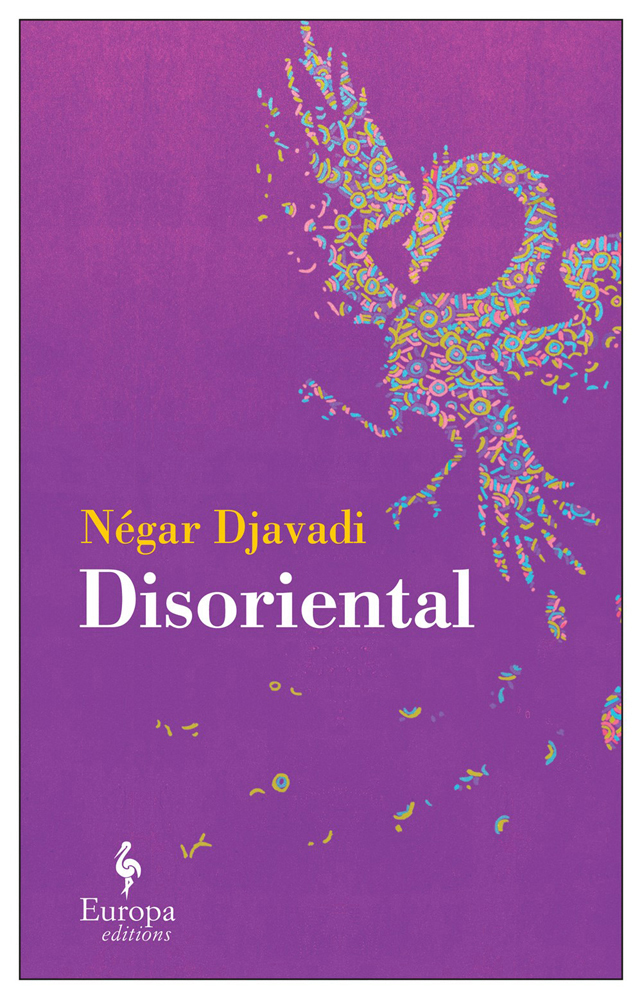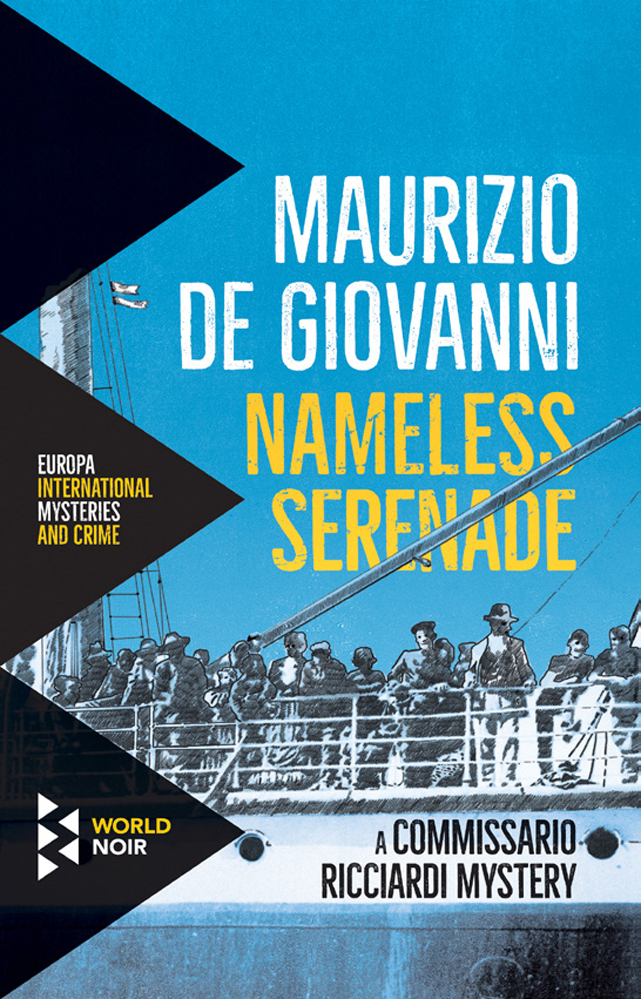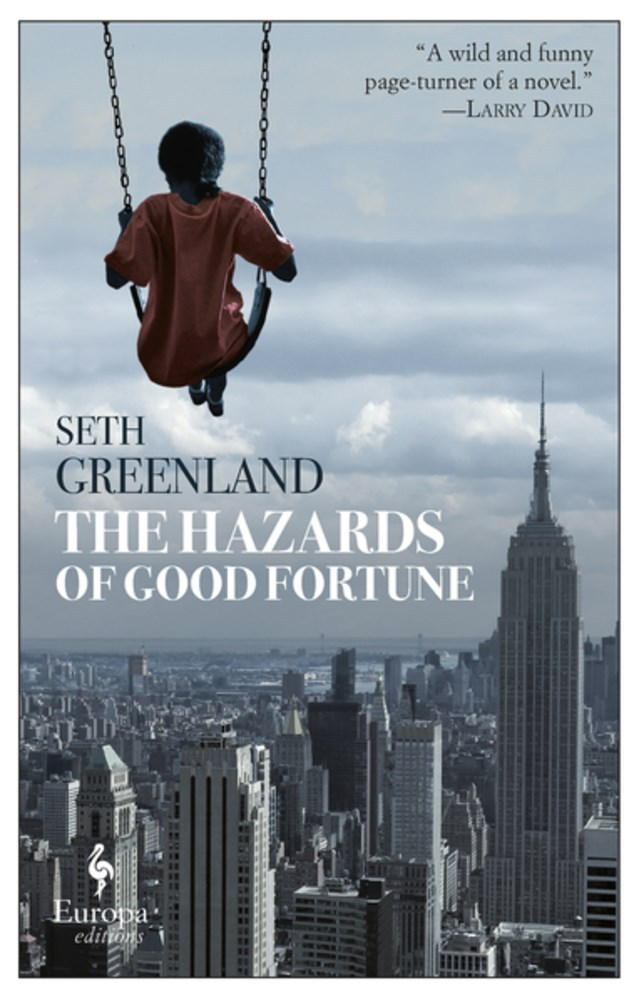/* styles */ Featured books: The Sacco Gang, £9.99; Disoriental, £12.99; Nameless Serenade, £12.99 & The Hazards of Good Fortune, £14.99 The Sacco Gang was included in the Sunday Times' foreign fiction round-up here, while Negar Djavadi's Disoriental was reviewed in The Guardian. Nameless Serenade has been included in a giveaway by The Times Crime Club, alongside the other novels in the Commissario Ricciardi series. The Hazards of Good Fortune was included in the second part of A Life in Books' 'Books to Look Out For in August' list.
 table div table+table+table+table+table+table+table+table+table+table+table+table+table+table+table+table+table+table+table+table+table+table+table+table+table+table+table div table{width:100%;padding:0}table div table+table+table+table+table+table+table+table+table+table+table+table+table+table+table+table+table+table+table+table+table+table+table+table+table+table+table div table img{width:96.23%;padding:0;float:none}table div table+table+table+table+table+table+table+table+table+table+table+table+table+table+table+table+table+table+table+table+table+table+table+table+table+table+table div table td{width:100%;padding:0 1.88% 18px}/* styles */## Melville House UK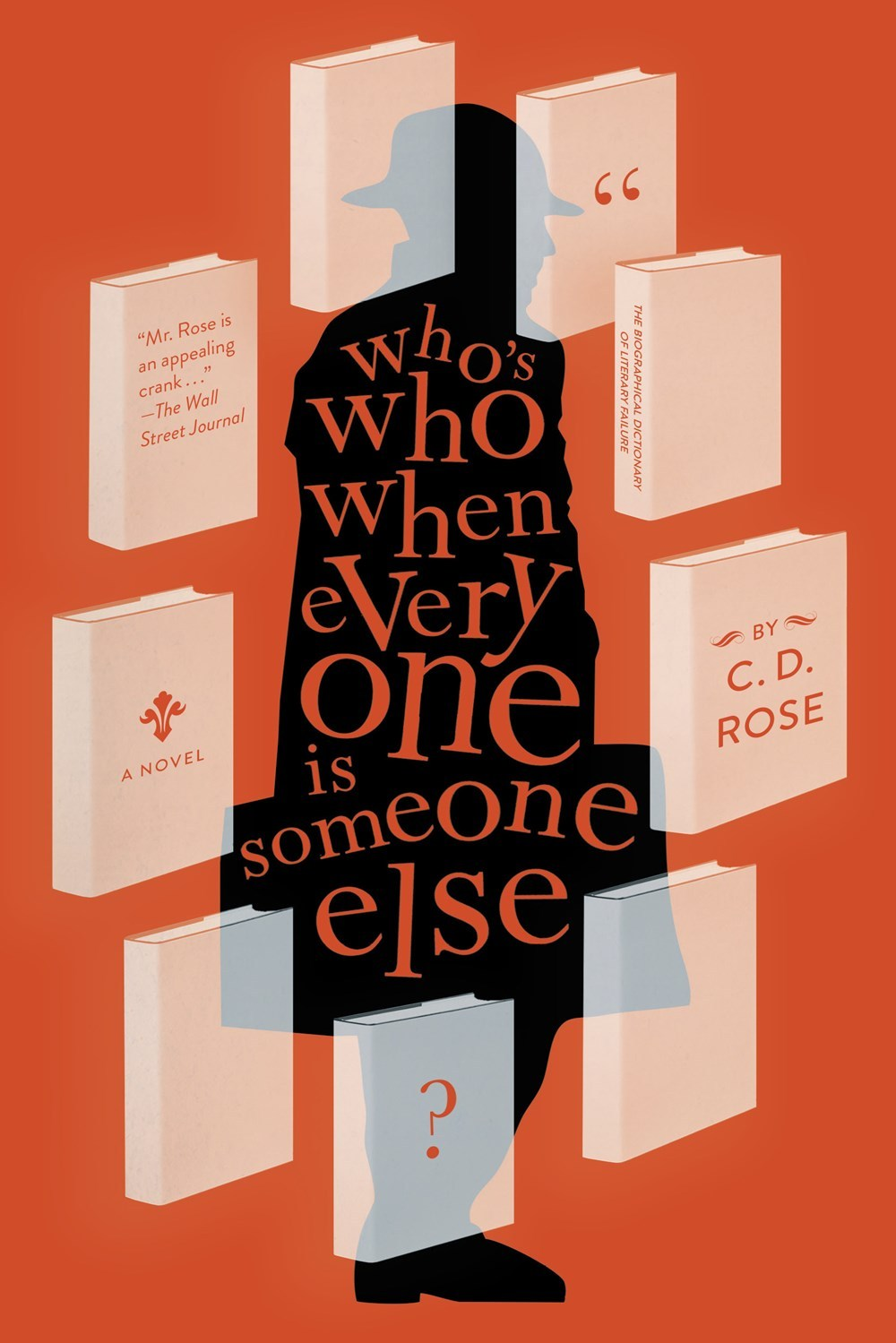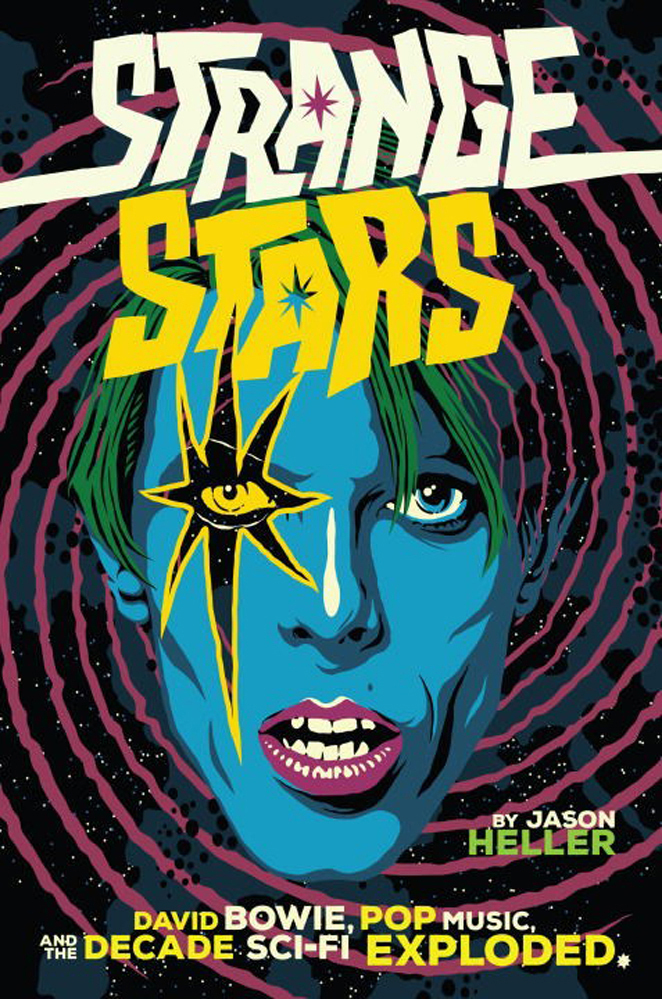/* styles */ Featured books: Who's Who When Everyone is Someone Else?, £12.99 & Strange Stars, £20.00 Who's Who When Everyone is Someone Else? was reviewed in TLS with the writer calling the novel 'ingenious, uproarious'. Meanwhile, Aquarium Drunkard's article on Strange Stars was shared on the Brian Eno Twitter feed.
 table div table+table+table+table+table+table+table+table+table+table+table+table+table+table+table+table+table+table+table+table+table+table+table+table+table+table+table+table+table+table+table div table{width:100%;padding:0}table div table+table+table+table+table+table+table+table+table+table+table+table+table+table+table+table+table+table+table+table+table+table+table+table+table+table+table+table+table+table+table div table img{width:96.23%;padding:0;float:none}table div table+table+table+table+table+table+table+table+table+table+table+table+table+table+table+table+table+table+table+table+table+table+table+table+table+table+table+table+table+table+table div table td{width:100%;padding:0 1.88% 18px}/* styles */## Downtown Bookworks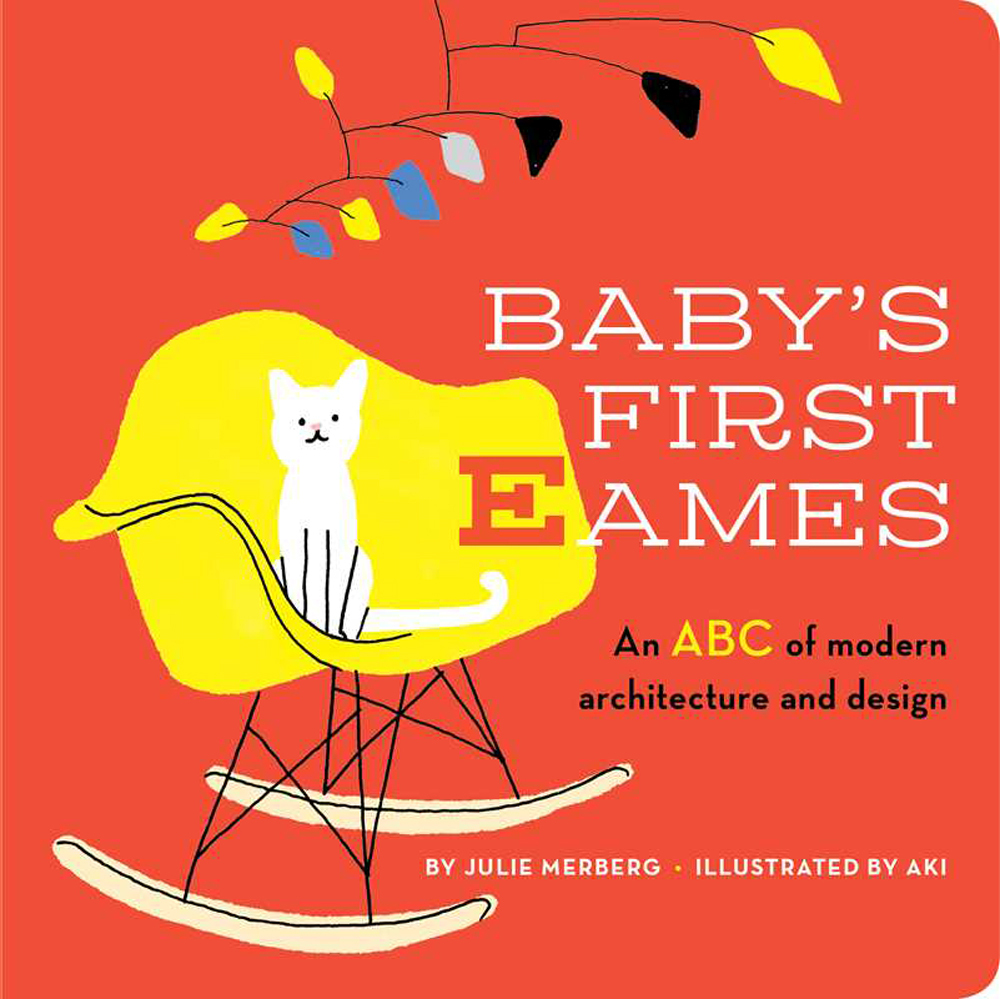Featured book: Baby's First Eames, £9.99

Baby's First Eames has had a good run, with reviews in Dallas News, Little London Magazine, Young Architect, Architect Magazine and Apartment Therapy.

 table div table+table+table+table+table+table+table+table+table+table+table+table+table+table+table+table+table+table+table+table+table+table+table+table+table+table+table+table+table+table+table+table+table+table div table{width:100%;padding:0}table div table+table+table+table+table+table+table+table+table+table+table+table+table+table+table+table+table+table+table+table+table+table+table+table+table+table+table+table+table+table+table+table+table+table div table img{width:96.23%;padding:0;float:none}table div table+table+table+table+table+table+table+table+table+table+table+table+table+table+table+table+table+table+table+table+table+table+table+table+table+table+table+table+table+table+table+table+table+table div table td{width:100%;padding:0 1.88% 18px}/* styles */## New InternationalistFeatured book: 80-20: Development in an Unequal World, £20.00

80-20: Development in an Unequal World was called 'an asset for every busy geography teacher' by Teaching Geography.

## PM Press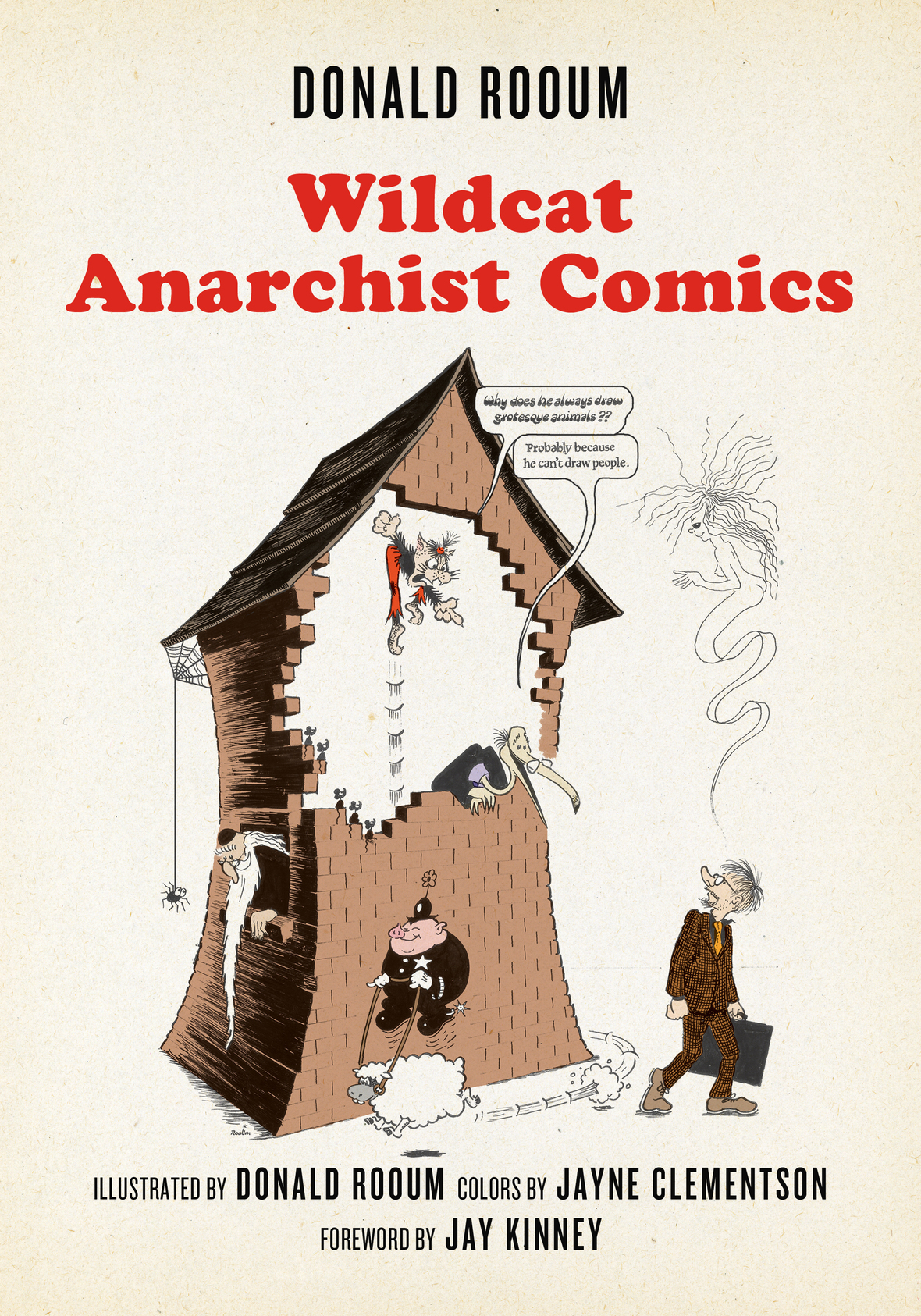/* styles */ Featured books: Health Care Revolt, £13.99 & Wildcat Anarchist Comics, £13.99 Health Care Revolt was reviewed in Publishers Weekly here, while Wildcat Anarchist Comics author Donald Rooum was interviewed on The Final Straw Radio here.
 table div table+table+table+table+table+table+table+table+table+table+table+table+table+table+table+table+table+table+table+table+table+table+table+table+table+table+table+table+table+table+table+table+table+table+table+table+table+table+table+table div table{width:100%;padding:0}table div table+table+table+table+table+table+table+table+table+table+table+table+table+table+table+table+table+table+table+table+table+table+table+table+table+table+table+table+table+table+table+table+table+table+table+table+table+table+table+table div table img{width:96.23%;padding:0;float:none}table div table+table+table+table+table+table+table+table+table+table+table+table+table+table+table+table+table+table+table+table+table+table+table+table+table+table+table+table+table+table+table+table+table+table+table+table+table+table+table+table div table td{width:100%;padding:0 1.88% 18px}/* styles */## Dedalus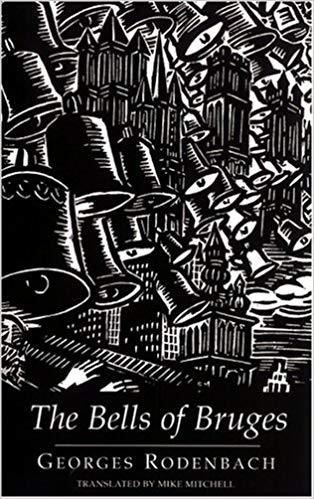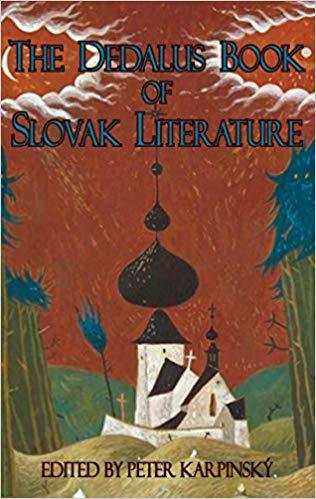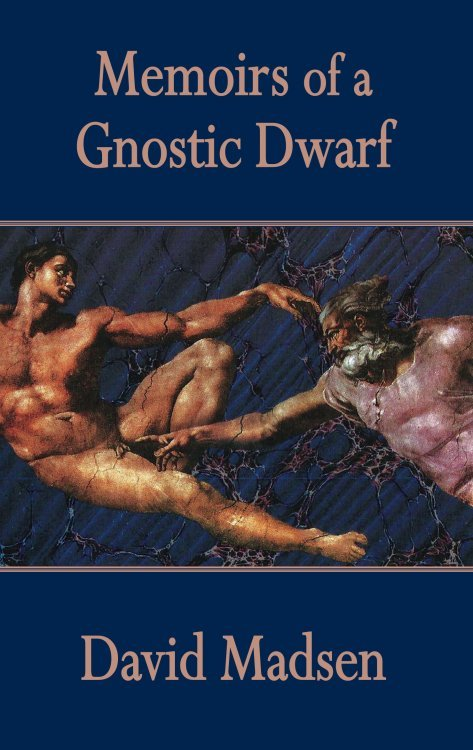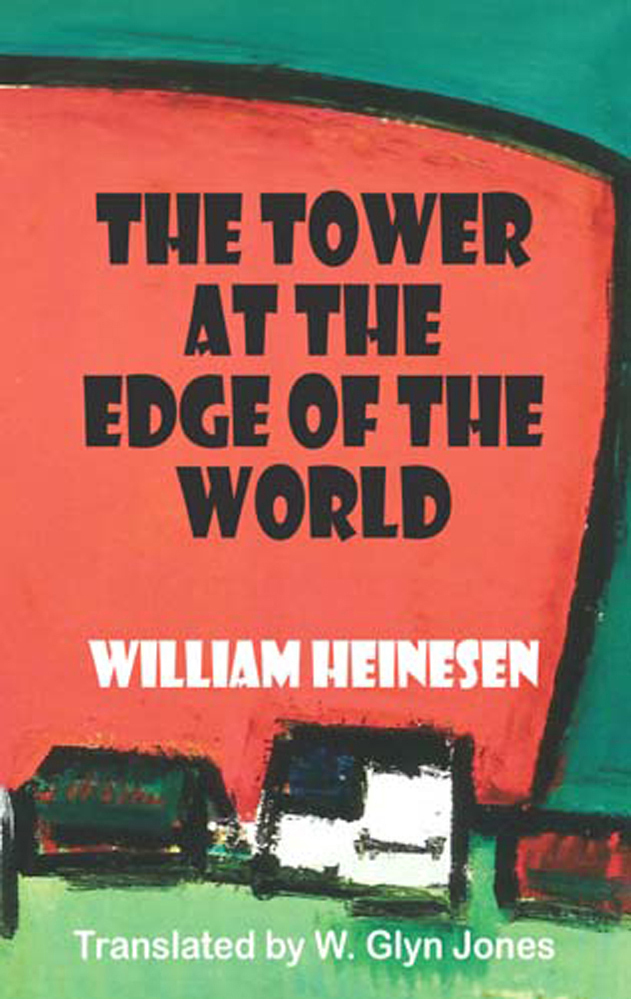/* styles */ Featured books: The Bells of Bruges, £9.99; The Dedalus Book of Slovak Literature, £11.99; Memoris of a Gnostic Dwarf, £9.99 & The Tower at the Edge of the World, £8.99 The Bells of Bruges was included in the second part of the Guardian's list of 'Best Summer Holiday Reads', while The Dedalus Book of Slovak Literature was chosen for Michael Dirda's summer reading in The Washington Post. Memoirs of a Gnostic Dwarf was reviewed on Christopher Fowler's blog, and several William Heinesen titles - including The Tower at the Edge of the World and The Black Cauldron - received a write up in the TLS.
 table div table+table+table+table+table+table+table+table+table+table+table+table+table+table+table+table+table+table+table+table+table+table+table+table+table+table+table+table+table+table+table+table+table+table+table+table+table+table+table+table+table+table+table+table div table{width:100%;padding:0}table div table+table+table+table+table+table+table+table+table+table+table+table+table+table+table+table+table+table+table+table+table+table+table+table+table+table+table+table+table+table+table+table+table+table+table+table+table+table+table+table+table+table+table+table div table img{width:96.23%;padding:0;float:none}table div table+table+table+table+table+table+table+table+table+table+table+table+table+table+table+table+table+table+table+table+table+table+table+table+table+table+table+table+table+table+table+table+table+table+table+table+table+table+table+table+table+table+table+table div table td{width:100%;padding:0 1.88% 18px}/* styles */## Bitter Lemon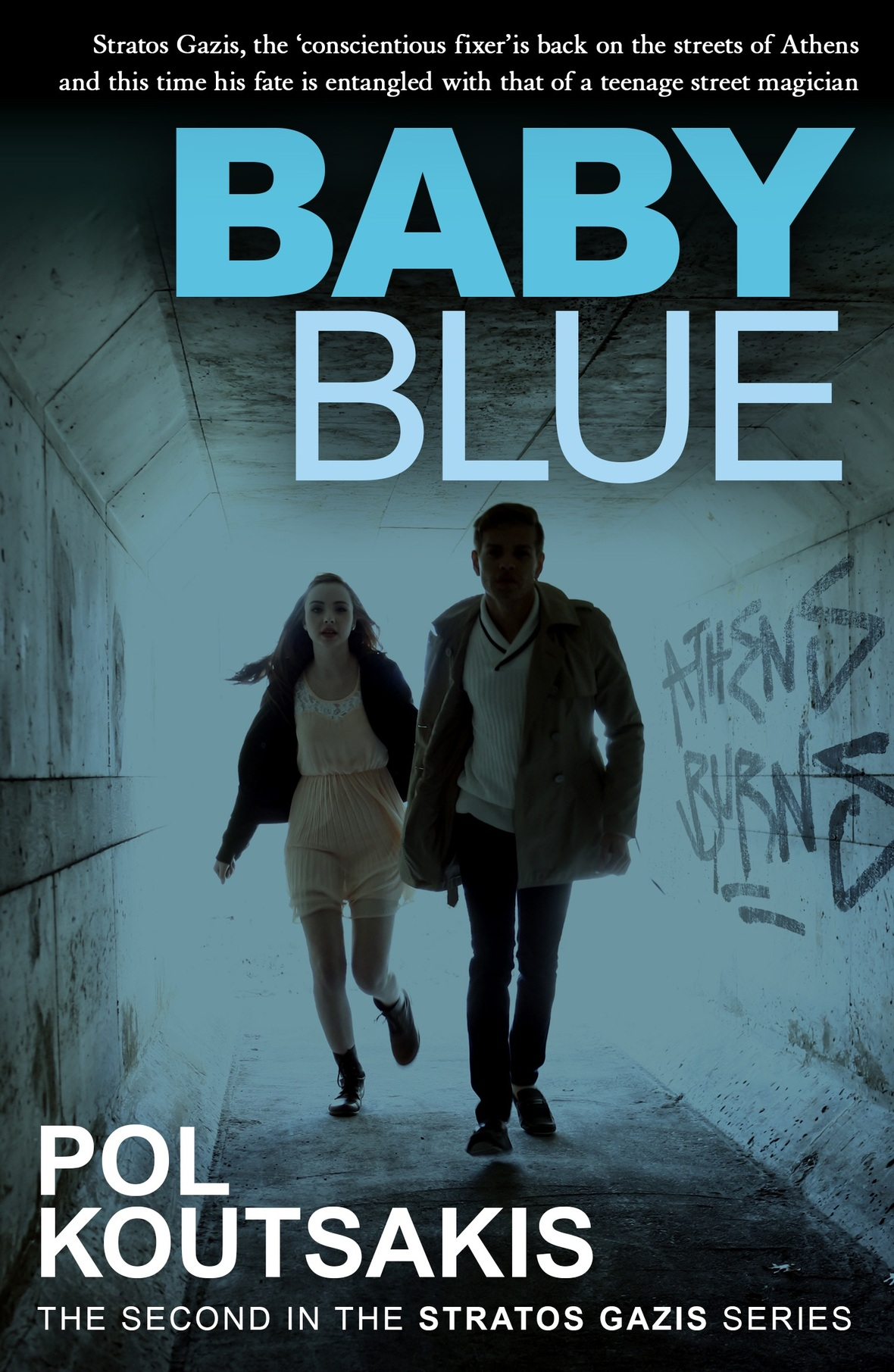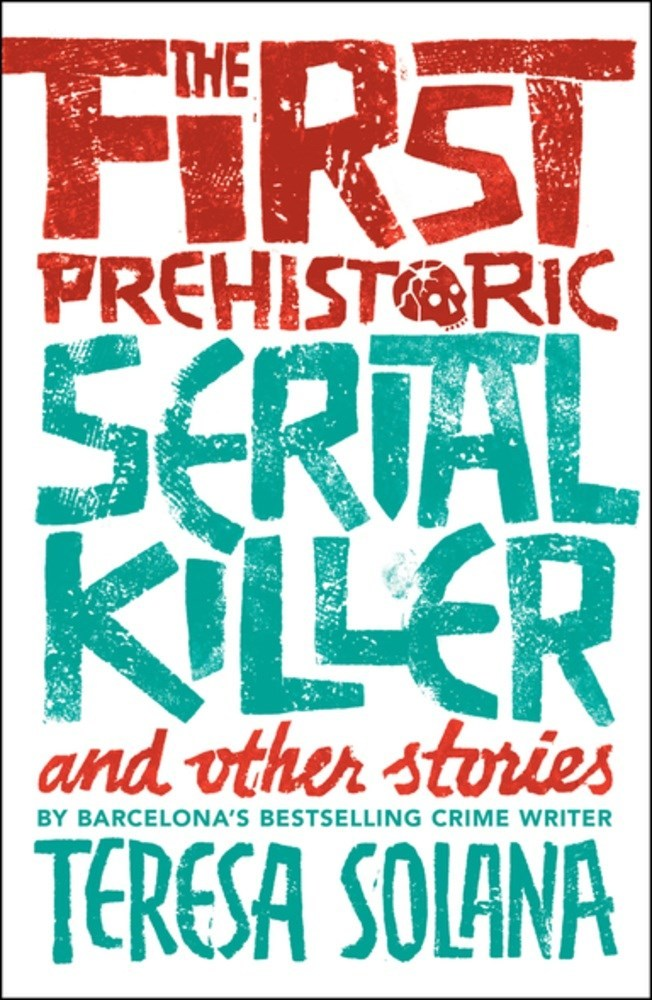/* styles */ Featured books: Baby Blue, £8.99 & The First Prehistoric Serial Killer, £8.99 Baby Blue and The First Prehistoric Serial Killer were both included in Dedalus Books' list of Summer Reading from Independent Publishers here.
 table div table+table+table+table+table+table+table+table+table+table+table+table+table+table+table+table+table+table+table+table+table+table+table+table+table+table+table+table+table+table+table+table+table+table+table+table+table+table+table+table+table+table+table+table+table+table+table+table div table{width:100%;padding:0}table div table+table+table+table+table+table+table+table+table+table+table+table+table+table+table+table+table+table+table+table+table+table+table+table+table+table+table+table+table+table+table+table+table+table+table+table+table+table+table+table+table+table+table+table+table+table+table+table div table img{width:96.23%;padding:0;float:none}table div table+table+table+table+table+table+table+table+table+table+table+table+table+table+table+table+table+table+table+table+table+table+table+table+table+table+table+table+table+table+table+table+table+table+table+table+table+table+table+table+table+table+table+table+table+table+table+table div table td{width:100%;padding:0 1.88% 18px}/* styles */## deCoubertin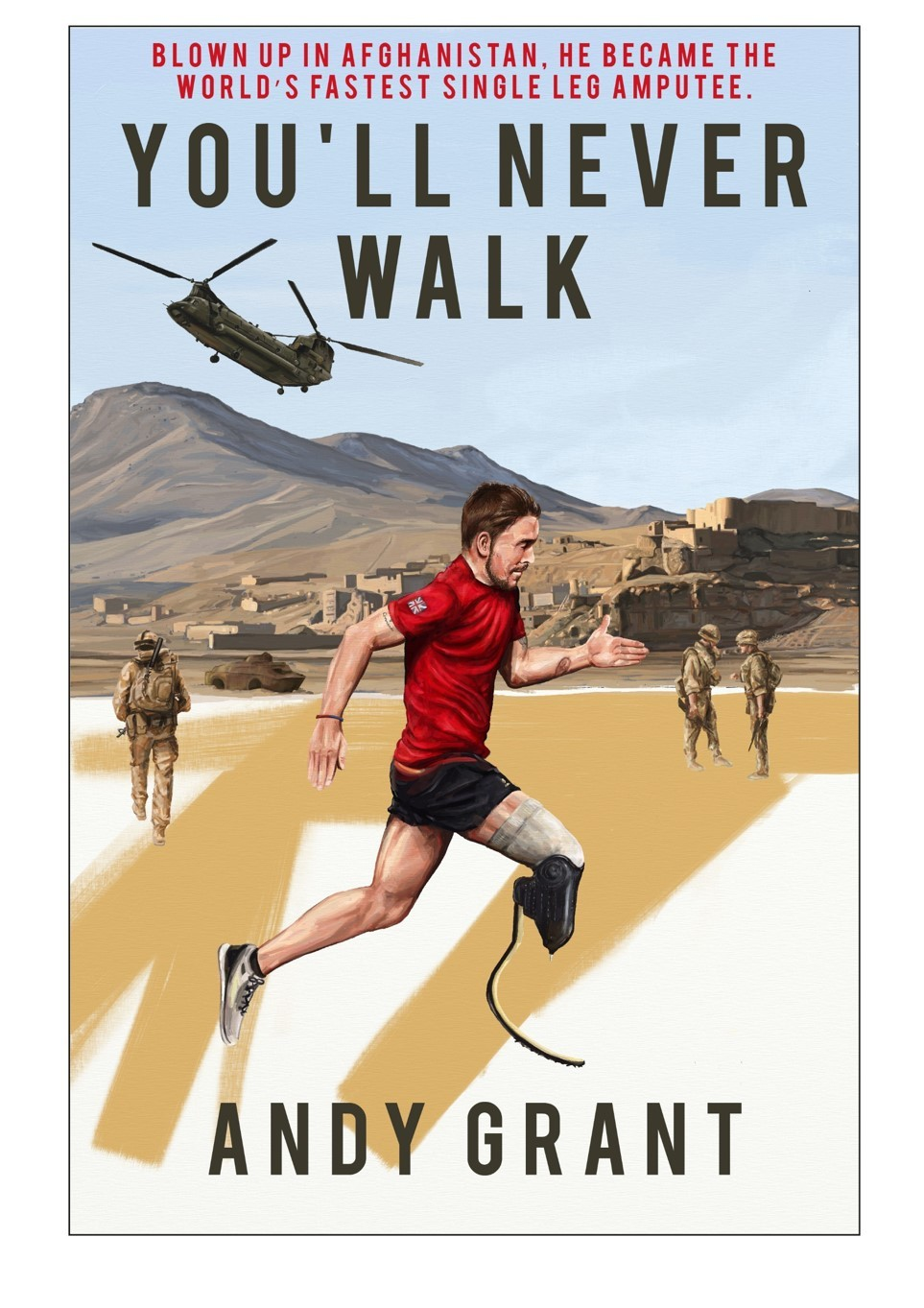/* styles */ Featured books: You'll Never Walk, £15.99 & There to be Shot At, £20.00 Andy Grant was interviewed by The Maelstrom recently, while When Saturday Comes reviewed There to be Shot At here.
 table div table+table+table+table+table+table+table+table+table+table+table+table+table+table+table+table+table+table+table+table+table+table+table+table+table+table+table+table+table+table+table+table+table+table+table+table+table+table+table+table+table+table+table+table+table+table+table+table+table+table+table+table div table{width:100%;padding:0}table div table+table+table+table+table+table+table+table+table+table+table+table+table+table+table+table+table+table+table+table+table+table+table+table+table+table+table+table+table+table+table+table+table+table+table+table+table+table+table+table+table+table+table+table+table+table+table+table+table+table+table+table div table img{width:96.23%;padding:0;float:none}table div table+table+table+table+table+table+table+table+table+table+table+table+table+table+table+table+table+table+table+table+table+table+table+table+table+table+table+table+table+table+table+table+table+table+table+table+table+table+table+table+table+table+table+table+table+table+table+table+table+table+table+table div table td{width:100%;padding:0 1.88% 18px}/* styles */## Event Diary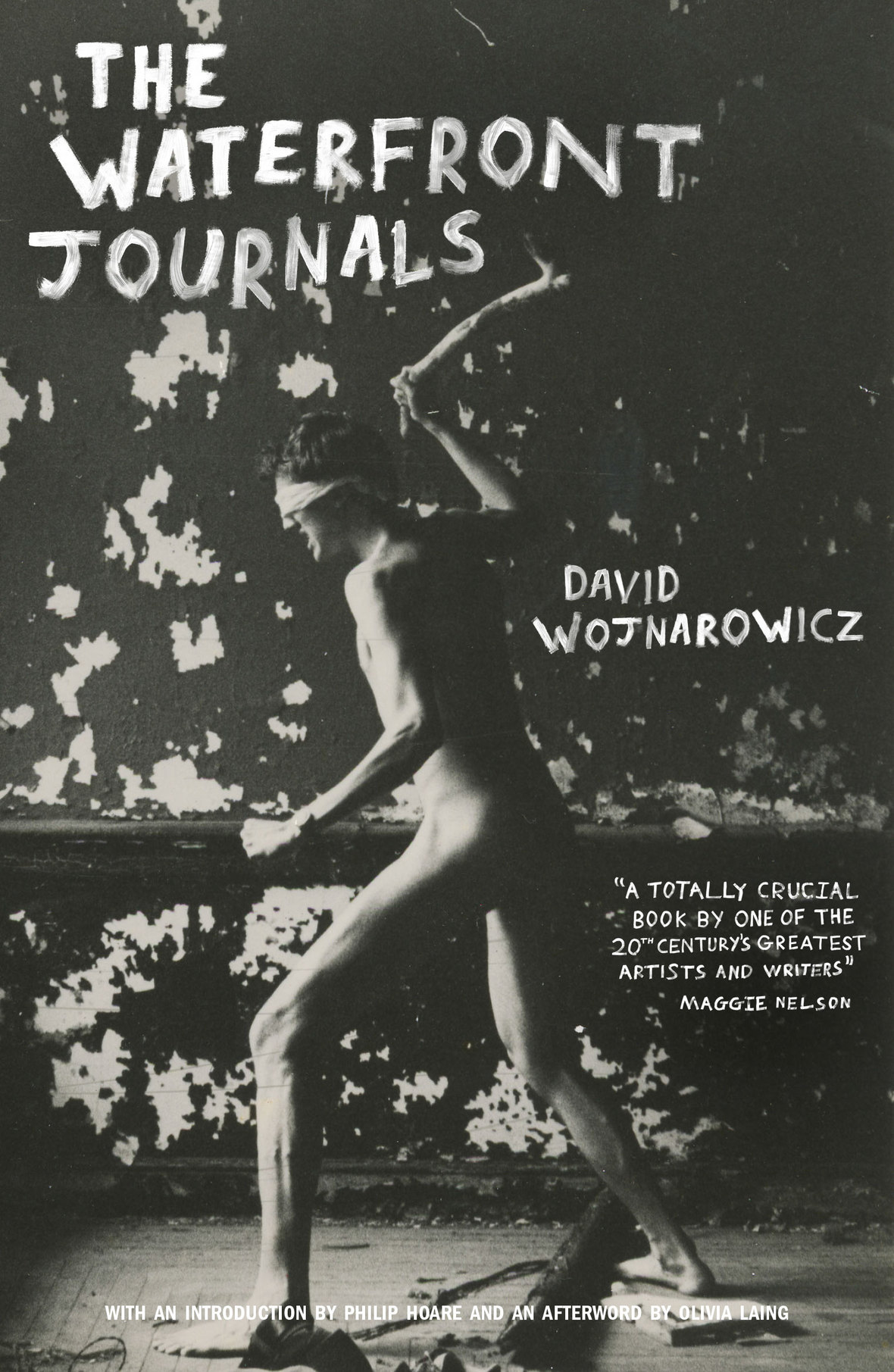# The Waterfront Journals Launch

## Thursday 2nd August at Burley Fisher Books.# Michael Redhill at Edinburgh Book Festival

## 2pm, 15th August at Baillie Gifford Corner Theatre.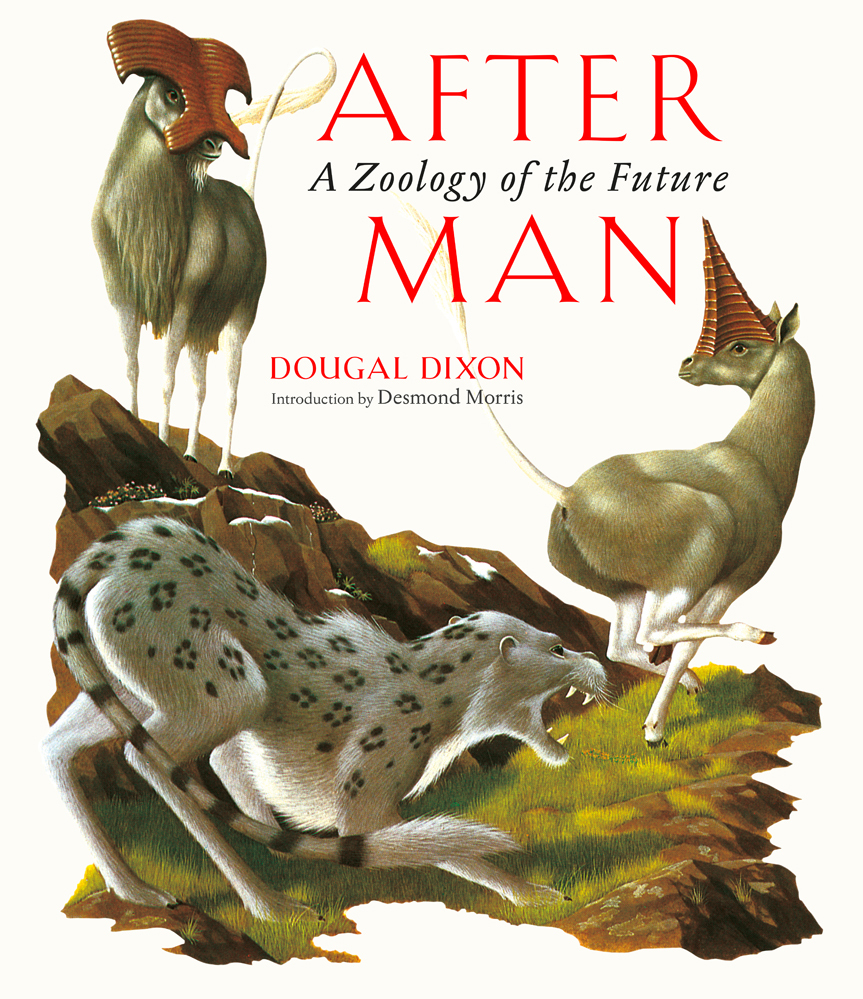# Dougal Dixon at The Conway Hall

## 11th September

Dougal will be speaking about his book After Man.

 table div table+table+table+table+table+table+table+table+table+table+table+table+table+table+table+table+table+table+table+table+table+table+table+table+table+table+table+table+table+table+table+table+table+table+table+table+table+table+table+table+table+table+table+table+table+table+table+table+table+table+table+table+table+table+table+table+table div table{width:100%;padding:0}table div table+table+table+table+table+table+table+table+table+table+table+table+table+table+table+table+table+table+table+table+table+table+table+table+table+table+table+table+table+table+table+table+table+table+table+table+table+table+table+table+table+table+table+table+table+table+table+table+table+table+table+table+table+table+table+table+table div table img{width:96.23%;padding:0;float:none}table div table+table+table+table+table+table+table+table+table+table+table+table+table+table+table+table+table+table+table+table+table+table+table+table+table+table+table+table+table+table+table+table+table+table+table+table+table+table+table+table+table+table+table+table+table+table+table+table+table+table+table+table+table+table+table+table+table div table td{width:100%;padding:0 1.88% 18px}/* styles */